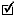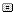#Name Topic Used in Type
valueStates numberattribute
The value of the number, like "50". You can use operators like "+" and "-" to handle calculations. For example, "10 + [a] * (2 / [b])" is a valid calculation.

Number operators
OperatorDescriptionUsage
+ Sum a number value="[a] + 4"
- Subtract a number value="[a] - 3"
* Multiply a number value="[a] * [b]"
/ Divide a number value="[a] / 2"
^ Raise a number to the power of an exponent value="[a] ^ 2"
Sample:
``` <text>You see a big treasure and stuff yourself   with 50 gold coins. </text> <state name="treasureChestEmpty" /> <number name="gold" value="[gold] + 50" /> <choice station="back">Close treasure chest</choice> ```